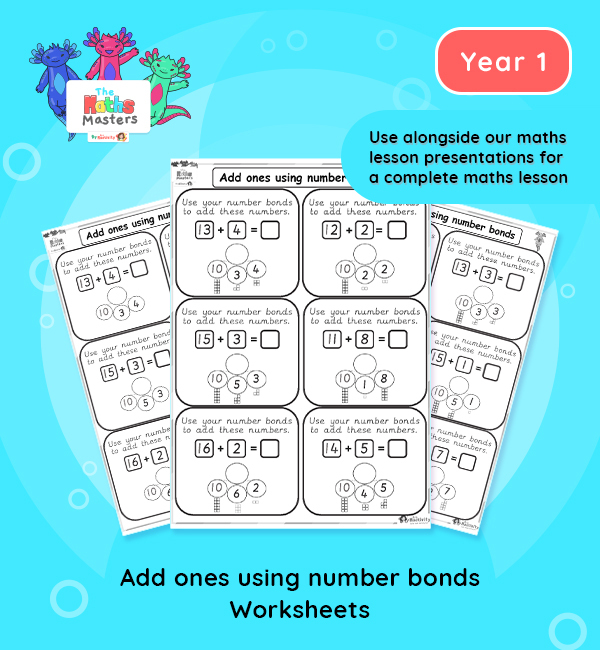# Year 1 | Adding Ones Using Number Bonds Worksheet## Year 1 addition and subtraction worksheets

Aligned with the maths mastery approach, these Year 1 | Adding Ones Using Number Bonds Worksheet is designed to be used alongside our Year 1 | Adding Ones Using Number Bonds Lesson Presentation and requires children to use their knowledge of part part whole models, and pictorial representations, to do simple number bond additions.

Small Steps: Add ones using number bonds.

NC Links: Represent and use number bonds and related subtraction facts within 20. * Read, write and interpret mathematical statements involving addition, subtraction and equals signs. *Add and subtract one-digit and two-digit numbers to 20, including zero.

TAF Statements: Working towards: Add and subtract (one digit numbers) explaining their method verbally, in pictures, or using apparatus.

Working At: Recall all the number bonds to and within 10 and use these to reason with and calculate bonds to and within 20, recognising other associated additive relationships.

Greater Depth – Use reasoning about number and relationships to solve more complex problems and explain their thinking.

Ready-to-progress criteria:  1NF-1 Develop fluency in addition and subtraction facts within 10

Future applications: Add and subtract across 10.

1AS-2 Read, write and interpret equations containing addition(+), subtraction (-), and equals (=) symbols, and relate additive expressions and equations to real-life contexts.

## Recently Viewed